# Solve the following system of equations graphically:

Question:

Solve the following system of equations graphically:

Shade the region between the lines and the y-axis

(i) $3 x-4 y=7$

$5 x+2 y=3$

(ii) $4 x-y=4$

$3 x+2 y=14$

Solution:

The given equations are:

$3 x-4 y=7$$. .(i) 5 x+2 y=3$$. .(i i)$

Putting $x=0$ in equation (i) we get:

$\Rightarrow 3 \times 0-4 y=7$

$\Rightarrow y=-7 / 4$

$x=0, \quad y=-7 / 4$

Putting $y=0$ in equation (i) we get:

$\Rightarrow 3 x-4 \times 0=7$

$\Rightarrow x=7 / 3$

$x=7 / 3, \quad y=0$

Use the following table to draw the graph.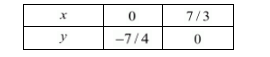Draw the graph by plotting the two points $A(0,-7 / 4), B(7 / 3,0)$ from table.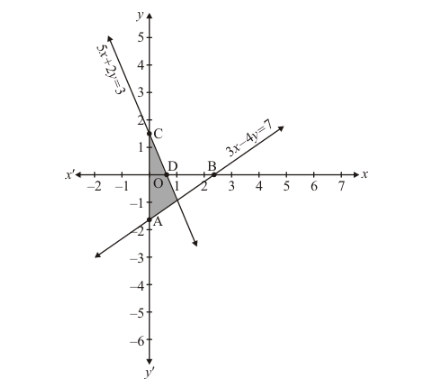$5 x+2 y=3$.(ii)

Putting $x=0$ in equation (ii) we get:

$\Rightarrow 5 \times 0+2 y=3$

$\Rightarrow y=2 / 3$

$x=0, \quad y=3 / 2$

Putting $y=0$ in equation (ii) we get:

$\Rightarrow 5 x+2 \times 0=3$

$\Rightarrow x=3 / 5$

$x=3 / 5, \quad y=0$

Use the following table to draw the graph.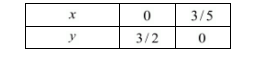Draw the graph by plotting the two points $C(0,3 / 2)$ and $D(3 / 5,0)$ from table.

The two lines intersect at points $P(1,-1)$ of $y$-axis.

Hence, $x=1$ and $y=-1$ is the Solution.

(ii) The equations are:

$4 x-y=4$(1)

$3 x+2 y=14$$(2)$

Putting $x=0$ in equation (1) we get:

$\Rightarrow 4 \times 0-y=4$

$\Rightarrow y=-4$

$x=0, \quad y=-4$

Putting $y=0$ in equation (1) we get:

$\Rightarrow 4 x-0=4$

$\Rightarrow x=1$

$x=1, \quad y=0$

Use the following table to draw the graph: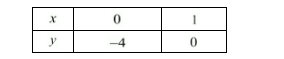Draw the graph by plotting the two points $A(0,-4)$ and $B(1,0)$ from table.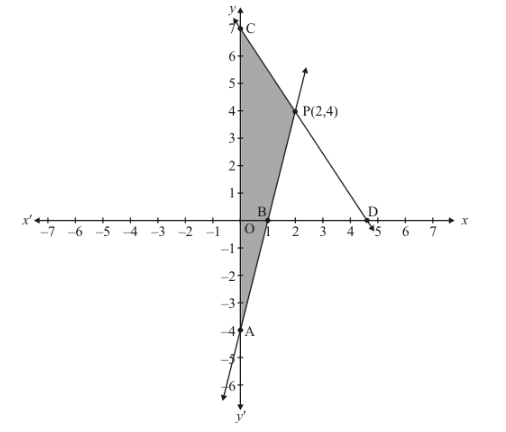$3 x+2 y=14$...(2)

Putting $x=0$ in equation (2) we get:

$\Rightarrow 3 \times 0+2 y=14$

$\Rightarrow y=7$

$x=0, \quad y=7$

Putting $y=0$ in equation (2) we get:

$\Rightarrow 3 x+2 \times 0=14$

$\Rightarrow x=14 / 3$

$x=14 / 3, \quad y=0$

Use the following table to draw the graph.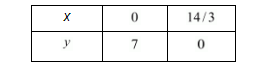Draw the graph by plotting the two points $C(0,7), D(14 / 3,0)$ from table.

Two lines intersect at points $P(2,4)$ of $y$-axis.

Hence $x=2$ and $y=4$ is the solution.